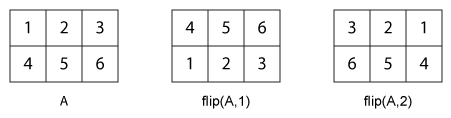# flip

## 语法

``B = flip(A)``
``B = flip(A,dim)``

## 说明

``B = flip(A)` 返回的数组 `B` 具有与 `A` 相同的大小，但元素顺序已反转。`B` 中重新排序的维度取决于 `A` 的形状：如果 `A` 为向量，`flip(A)` 将沿向量的长度方向反转元素顺序。如果 `A` 为矩阵，`flip(A)` 将反转每列元素的顺序。如果 `A` 为 N 维数组，`flip(A)` 将按 `A` 的大小值不等于 `1` 的首个维度上进行运算。`

``B = flip(A,dim)` 沿维度 `dim` 反转 `A` 中元素的顺序。例如，如果 `A` 为矩阵，`flip(A,1)` 将反转每一列中的元素，`flip(A,2)` 将反转每一行中的元素。`

## 示例

```A = 'no word, no bond, row on.'; B = flip(A)```
```B = '.no wor ,dnob on ,drow on' ```
```A = [1;2;3]; B = flip(A)```
```B = 3×1 3 2 1 ```

`A = diag([100 200 300])`
```A = 3×3 100 0 0 0 200 0 0 0 300 ```

`B = flip(A)`
```B = 3×3 0 0 300 0 200 0 100 0 0 ```

`B = flip(A,2)`
```B = 3×3 0 0 100 0 200 0 300 0 0 ```

```A = zeros(1,3,2); A(:,:,1) = [1 2 3]; A(:,:,2) = [4 5 6]; A```
```A = A(:,:,1) = 1 2 3 A(:,:,2) = 4 5 6 ```

`B = flip(A)`
```B = B(:,:,1) = 3 2 1 B(:,:,2) = 6 5 4 ```

`B = flip(A,3)`
```B = B(:,:,1) = 4 5 6 B(:,:,2) = 1 2 3 ```

`A = {'foo',1000; 999,true; 'aaa','bbb'}`
```A=3×2 cell array {'foo'} {} {} {[ 1]} {'aaa'} {'bbb' } ```

`B = flip(A)`
```B=3×2 cell array {'aaa'} {'bbb' } {} {[ 1]} {'foo'} {} ```

`B = flip(A,2)`
```B=3×2 cell array {} {'foo'} {[ 1]} {} {'bbb' } {'aaa'} ```

## 输入参数

• `flip(A,1)` 反转 `A` 的每列中元素的顺序，并返回一个 `m`×`n` 矩阵。

• `flip(A,2)` 反转 `A` 的每行中元素的顺序，并返回一个 `m`×`n` 矩阵。